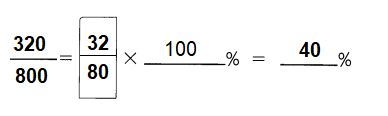# Math in Focus Grade 5 Chapter 10 Practice 2 Answer Key Expressing Fractions as Percents

Practice the problems of Math in Focus Grade 5 Workbook Answer Key Chapter 10 Practice 2 Expressing Fractions as Percents to score better marks in the exam.

## Math in Focus Grade 5 Chapter 10 Practice 2 Answer Key Expressing Fractions as Percents

Express each fraction as a percent.

Example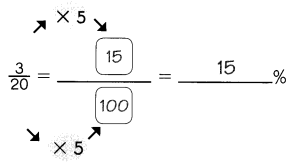Question 1.
$$\frac{26}{50}$$ = ______%
Explanation:
To convert a fraction to a percent, first multiply the numerator and the denominator with 5.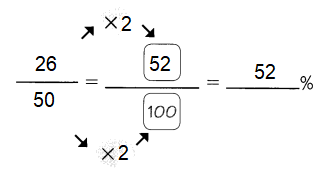Question 2.
$$\frac{4}{5}$$ = ______%
Explanation:
To convert a fraction to a percent, first multiply the numerator and the denominator with 25.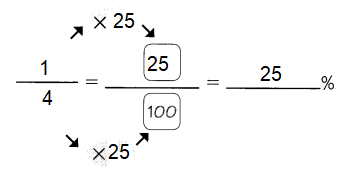Question 3.
$$\frac{19}{25}$$ = ______%
Explanation:
To convert a fraction to a percent, first multiply the numerator and the denominator with 4.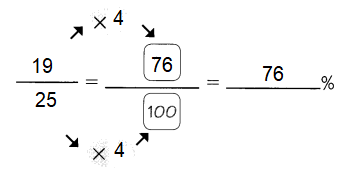Question 4.
$$\frac{1}{4}$$ = ______%
Explanation:
To convert a fraction to a percent, first multiply the numerator and the denominator with 25.Express each fraction as a percent.

Example
$$\frac{1}{5}$$ = $$\frac{1}{5}$$ × 100% = 20

Question 5.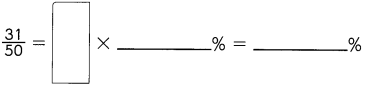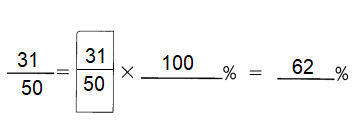Explanation:
To convert a fraction to a percent, first divide the numerator by the denominator.
Then multiply the decimal by 100 to express fraction as percentage.

Question 6.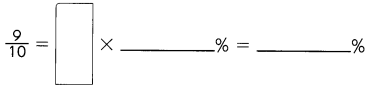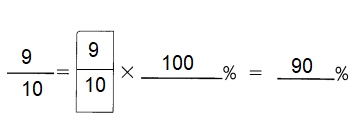Explanation:
To convert a fraction to a percent, first divide the numerator by the denominator.
Then multiply the decimal by 100 to express fraction as percentage.

Question 7.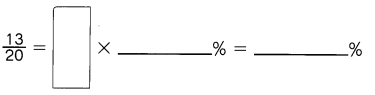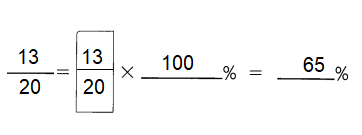Explanation:
To convert a fraction to a percent, first divide the numerator by the denominator.
Then multiply the decimal by 100 to express fraction as percentage.

Express each percent as a fraction in simplest form.

Example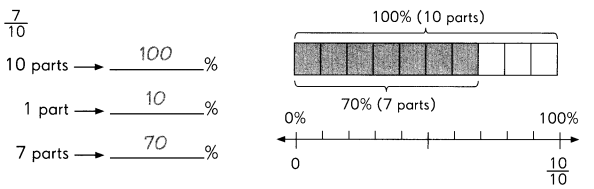Question 8.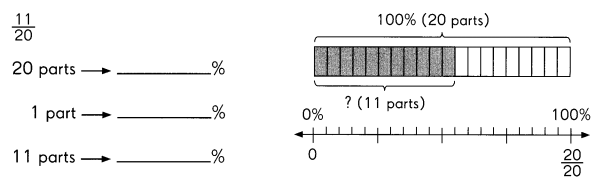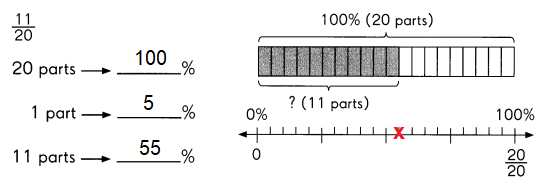Explanation:
To convert a percent to a fraction, we have to remove the percent sign and divide the given number by 100.
And, then we express the fractional form of the percentage in the simplest form.
Divide 100% into 20 parts as each part has 5%.
So, 11 parts is equal to 55%.

Question 9.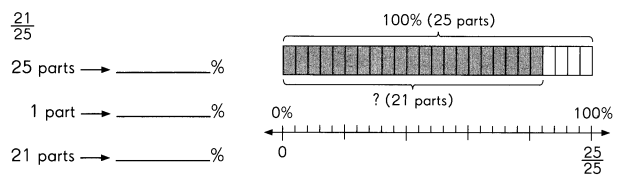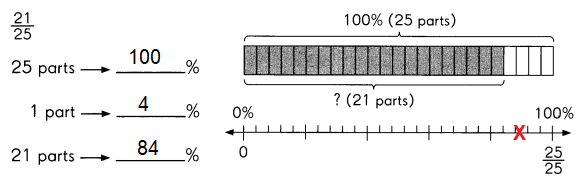Explanation:
To convert a percent to a fraction, we have to remove the percent sign and divide the given number by 100.
And, then we express the fractional form of the percentage in the simplest form.
Divide 100% into 25 parts as each part has 4%.
So, 21 parts is equal to 84%.

Express each fraction as a percent.

Question 10.
$$\frac{64}{200}$$ = $$\frac{32}{100}$$ = ___ %
Explanation:
To convert a fraction to a percent, first divide the numerator by the denominator.
Then multiply the answer by 100 .
It can be converted to percent by multiplying the decimal by 100 .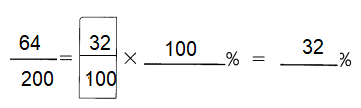Question 11.
$$\frac{1300}{400}$$ = ___ %
Explanation:
To convert a fraction to a percent, first divide the numerator by the denominator.
Then multiply the answer by 100 .
It can be converted to percent by multiplying the decimal by 100 .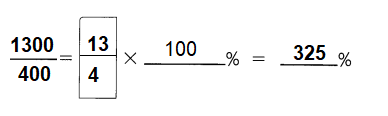Question 12.
$$\frac{480}{600}$$ = ___ %
Explanation:
To convert a fraction to a percent, first divide the numerator by the denominator.
Then multiply the answer by 100 .
It can be converted to percent by multiplying the decimal by 100 .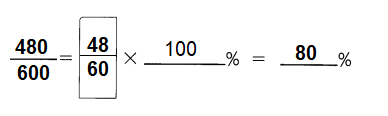Question 13.
$$\frac{518}{700}$$ = ___ %
Explanation:
To convert a fraction to a percent, first divide the numerator by the denominator.
Then multiply the answer by 100 .
It can be converted to percent by multiplying the decimal by 100 .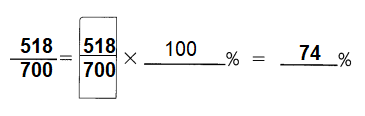Question 14.
Jeremy finished $$\frac{3}{5}$$ of his homework. What percent of his homework did he finish?Explanation:
To convert a fraction to a percent, first divide the numerator by the denominator.
Then multiply the answer by 100 .
It can be converted to percent by multiplying the decimal by 100 .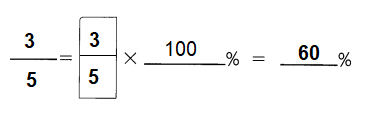Question 15.
Tracy ran in a marathon, but managed to complete only $$\frac{13}{20}$$ of the race.

a. What percent of the marathon did she complete?
Explanation:
To convert a fraction to a percent, first divide the numerator by the denominator.
Then multiply the answer by 100 .
It can be converted to percent by multiplying the decimal by 100 .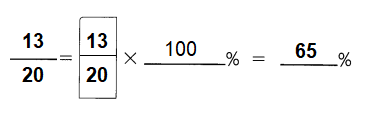b. Write each ratio as a fraction and then as a percent.
$$\frac{65}{100}$$ = 65 %
Explanation:
To convert a fraction to a percent, first multiply the numerator and the denominator with 5.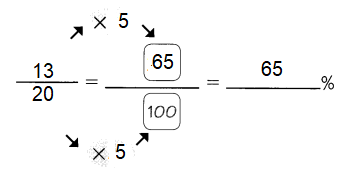Question 16.
Katie bought some flour. She used $$\frac{3}{8}$$ of it to bake bread. What percent of the flour is left?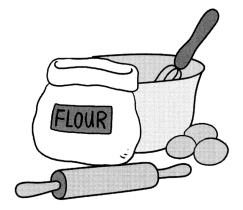Explanation: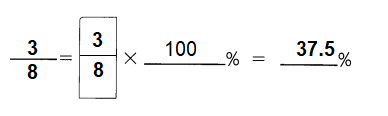She used $$\frac{3}{8}$$ of it to bake bread, it means 37.5%  used
100% – 37.5% = 62.5% of the flour is left.

Question 17.
There are 800 members in on astronomy club, and 320 of them are females. What percent of the members are males?